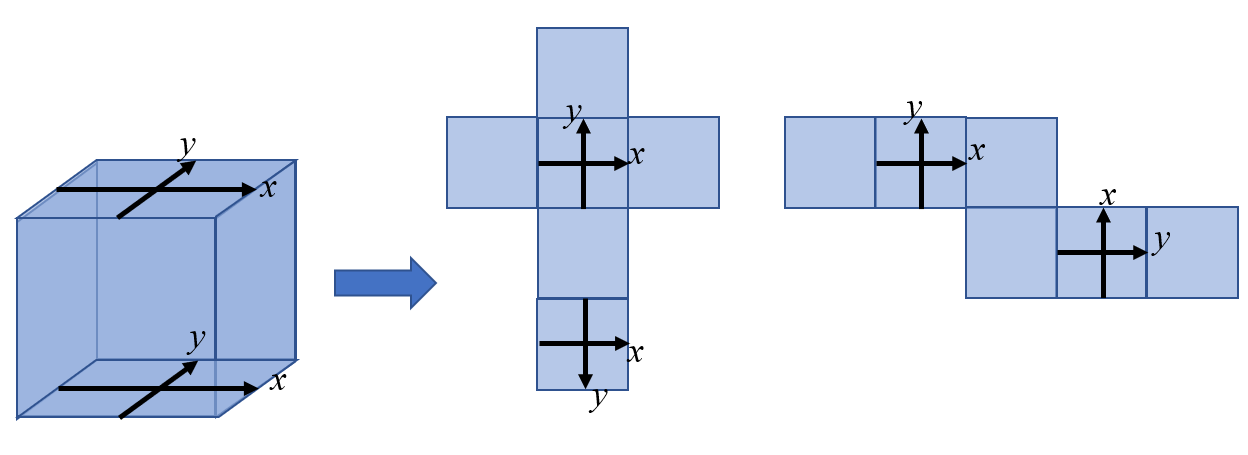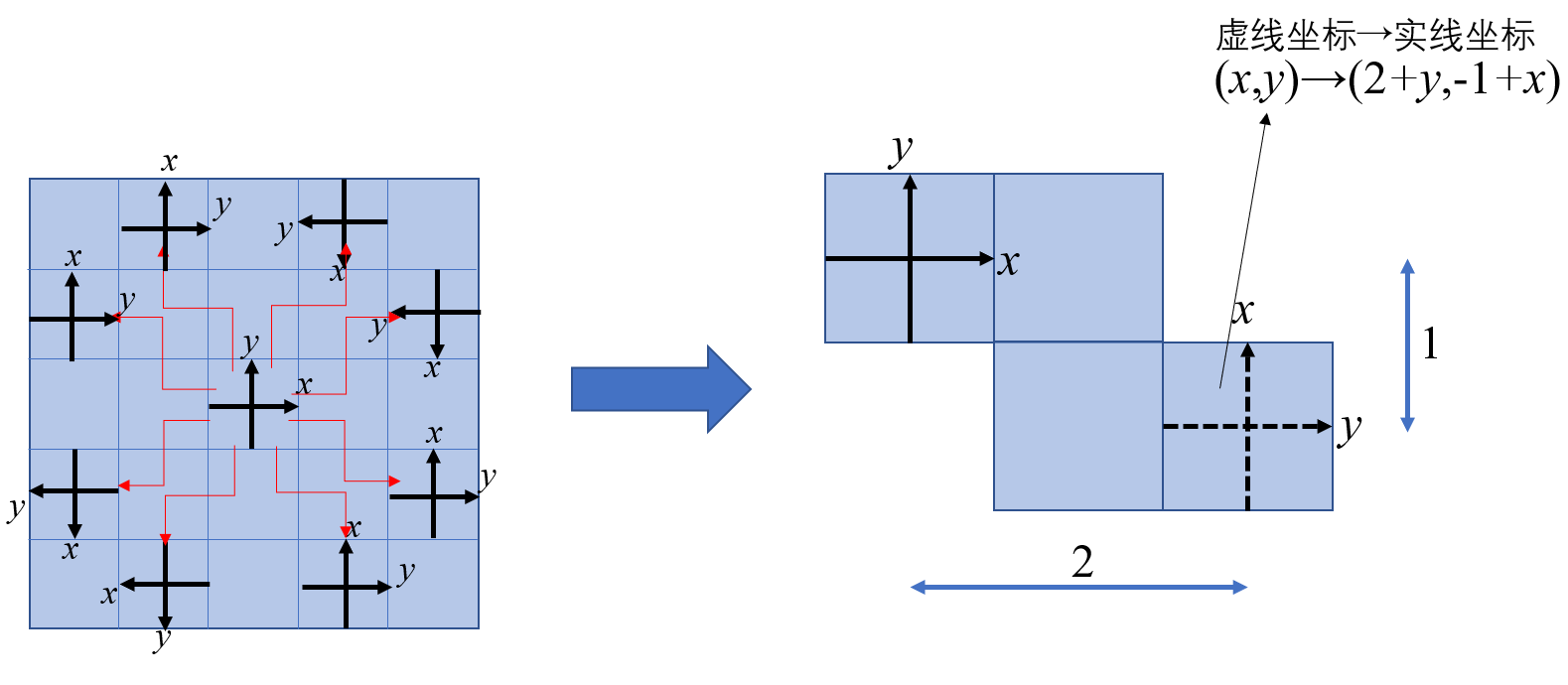# 洛谷 P2358 蚂蚁搬家 题解【枚举】【几何】

枚举+一定的空间想象力

## 输入输出格式

$4$个实数($-0.5$到$0.5$之间),分别表示出发点和目的点的坐标。

## 输入输出样例

0.26 0.50 0.50 0.18

1.146

## 题解：

一开始只考虑了四种情况，连样例都过不了。分析了一下样例发现可以经过$4$个面来达到最优解。用两个坐标系代表上下表面，正着的是上表面，则有这两种展开方式。左边那种比较好想，每次只有横坐标纵坐标在改变，枚举$4$种也比较好做；而右边这种实际上有$8$种情况，而且$x,y$轴都反过来了，还需要判断正方向。

好在还可以用坐标来表示，我们依然考虑在笛卡尔坐标系上求出两个点的欧几里得距离

说人话就是把坐标化在一个坐标系中，勾股定理求距离。

那么我们分别把这八种情况展开来，就是这样的：实线代表我们要统一的，也就是上表面的坐标系；虚线表示原来下表面的坐标系。

每种情况经过的四个面就是红色箭头经过的面。从中选出一个轨迹，把它折起来，对应的坐标系就变成了图上这样。

拿先右后下这条路径举个例子，由于$y$变横了，新的横坐标就是$2+y$，新的纵坐标是$-1+x$。其他路径的根据正方向所带的正负号不同。

上述一共$12$种情况，讨论完全就可以把这道题做出来了。

## Code：

#include<cstdio>
#include<cstring>
#include<cmath>
double x,y,x_,y_,ans=1e5,X,Y;
void upd()
{
double dis=sqrt((X-x)*(X-x)+(Y-y)*(Y-y));
ans=ans<dis?ans:dis;
}
int main()
{
scanf("%lf%lf%lf%lf",&x,&y,&x_,&y_);
X=2-x_;//右
Y=y_;
upd();
X=-2-x_;//左
upd();
X=x_;//上
Y=2-y_;
upd();
Y=-2-y_;//下
upd();

X=1-y_;//上右
Y=2-x_;
upd();
X=-1+y_;//上左
Y=2+x_;
upd();
X=-2+y_;//左上
Y=1+x_;
upd();
X=-2-y_;//左下
Y=-1-x_;
upd();
X=-1-y_;//下左
Y=-2-x_;
upd();
X=1+y_;//下右
Y=-2+x_;
upd();
X=2+y_;//右下
Y=-1+x_;
upd();
X=2-y_;//右上
Y=1-x_;
upd();
printf("%.3lf\n",ans);
return 0;
}

### 说点什么Subscribe

/* */